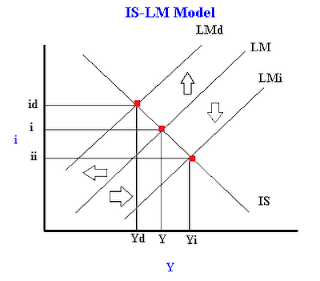What causes shifts in the IS or LM curves? - FreeEconHelp.com, Learning Economics... Solved!

## 8/14/18

This post was updated in August 2018 to include new information and examples.

This post goes over the economics and intuition of the IS/LM model and the possible causes for shifts in the two lines. It is possible for the IS curve (Investment and Savings) and the LM curve (Liquidity preference and Money supply) to either increase or decrease based on their determinants. Because of this, it is important to remember the two formulas for IS and LM, and that any change in the variables in the equations could cause a change in the graph:

The IS equation is:

Y = C(Y-T(Y))+I(r)+G+NX(Y)
Where
1. Y = national income or real GDP
2. C(Y-T(Y)) = consumption or consumer spending which is a function of disposable income
3. (Y-T(Y)) = disposable income which is equal to national income minus tax (which is a function of income)
4. I(r) = Investment which is a function of the real interest rate
5. G = Government spending/expenditures
6. NX(Y) = Net exports, where imports depend on income (Y)
Any change in the above variables can cause a shift in the IS curve, below we go through a case by case basis for each of the variables and give an example of how the IS curve is affected.

Possible shifts in the IS curve:

Change in Fiscal policy (G):When the IS curve shifts in the IS-LM model
1. The government can either increase government spending (shifting IS right) or decrease government spending (shifting IS left).
2. The government can also increase taxes which lowers consumer spending (shifting IS left) or decrease taxes which increases consumer spending (shifting IS right).

Consumers can change their savings rate (C):
1. If consumers decide to save more (which means that marginal propensity to consume declines) then consumer spending declines and the IS curve shifts left.
2. If consumers decide to save less (so marginal propensity to consume rises) then consumer spending increases and the IS curve shifts right.

Change in exports (NX):
1. If exports increase (normally due to currency depreciation) we will see the IS curve shift right.
2. If exports decrease (due to currency appreciation) we will see the IS curve shift left.
3. Important Note: Imports are endogenous in the model (they are a function of Y) so generally change in imports has no effect on the IS curve.  However, depending on your professor, a change in imports will have the opposite effect on the IS curve that exports has, so a decrease in imports shifts IS right, and an increase shifts IS left.
A change in private fixed investment (I)
1. If consumers/firms feel more confident about the future, they may invest more regardless of the interest rate.  This will cause an increase in investment (IS shifts right).
2. If consumers/firms feel less confident about the future, they may invest less regardless of the interest rate.  This will cause a decrease in investment (IS shifts left).

The LM (Liquidity preference and money supply equilibrium) formula:

M/P = L(i,Y)

Where
1. M/P = the real money supple where M is the actual amount of money in the economy and P is the overall price level
2. L(i,Y) = the real demand for money which is a function of the interest rate (i) and national income (Y)

Possible shifts in the LM curve:

A change in money supply (M):
1. If the central bank (or Federal Reserve) decides to increase the money supply (by buying t bills) then the LM curve shifts right.
2.When the LM curve shifts in the IS-LM Model
3. If the central bank (or Federal Reserve) decides to decrease the money supply (by selling t bills) then the LM curve shifts left.

A change in transaction technologies, ie credit cards (L):
1. If improvement in the velocity of money occurs such that people require less money to conduct all of their transactions, the LM curve will shift right (because the opportunity cost of holding money goes down because there is now an alternative).

A change in the overall price level (P):
1. If the price level rises, the LM curve shifts left.  This occurs because people need more money to pay the higher prices, but the higher resulting interest rates lower the demand for money.
2. If the price level declines, the LM curve shifts right.  This occurs because people need less money to pay the lower prices, and the lower interest rates increase their demand for holding money.
This post has shown all of the possible reasons for shifts in the IS or LM curves to occur. When a shift occurs in the IS/LM Model you need to figure out the direction of the shift, and then find the new equilibrium point to see what direction the change in equilibrium interest rate and GDP will be. Depending on the cause of the shift, we may see the new interest rate increase or decrease and the new GDP level may do the same. For more examples or if you would like to solidify your intuitive understanding of how the IS LM curves can shift please check out the video tutorial below: